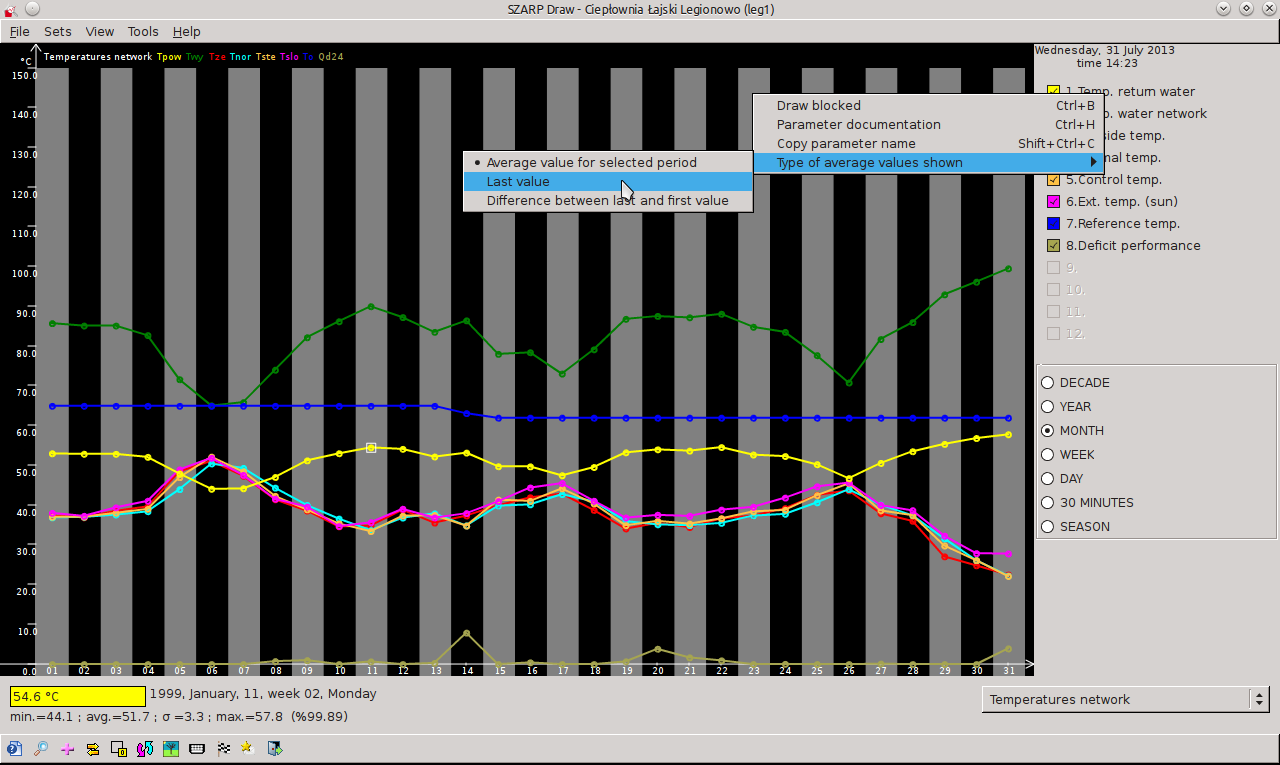# 3.15. Changing the type of the value represented by the point

The standard by every point on the graph corresponds to the average value parameter from the time represented by this point. This can be changed so to each point corresponds to:

• The final value of the period represented by the point (like the Annual screen each point will represent the value of the parameter of last day of the month 23:50 hours)

• The difference between the last and the first value in the 10-minute sample for the period represented by point (e.g., for a year will be the difference between the from 23:50 on the last day of the month and 0:00 of the first day of the month)

Change this setting to make the context menu right click on this parameter in the parameter list. Main sense of the existence of this option is that for some parameters more interesting from that which was the average value of a parameter in a given period, This is the value accepted this parameter, eg at the end of the month or provided that in the period parameter value has increased. This is particularly useful for parameters representing different kinds of counters.Rysunek 3-22. Changing the type of the value represented by the point